Univariate Descriptive Statistics

• "Statistics" is four things:
• An academic subject or discipline. Department of Statistics
• A set of methods you use to see patterns in the data
• Collections of data gathered with the methods described above
• A set of figures that summarize samples
• Descriptive and Inferential Statistics
• Recently you learned what samples are about the relation between samples and their populations.
• The statistical methods used for samples are different from the ones used for populations.
• The ones used for samples are descriptive statistics.
• The ones used for populations are inferential statistics.
• Descriptive statistics are simple
• Their purpose is to describe the data in a sample.
• They do this by replacing the dozens, hundreds, or thousands of numbers in your data with simple, easy-to-comprehend summaries of the numbers in your data.
• What is the most common value?
• What is the most central value?
• What is the average?
• How spread out are the values?
• Inferential statistics are more complicated
• Where you would use descriptive statistics to summarize the data from your sample, you don't have data from the population, so you have to do something different.
• You use inferential statistics to make estimates about the population -- the most common value, the most central value, etc.
• The math is more complicated than for descriptive statistics,
• but it is the logic involved that you are more likely to find confusing.

 Descriptive statistics vs. Inferential statistics You use descriptive statistics when you want to describe a sample You use inferential statistics to make estimates about a population Descriptive statistics describe or summarize the data in a sample Inferential statistics are estimates that describe or summarize the data in a population.

• How many variables?
• univariate statistics: one variable
• bivariate statistics: two variables
• multivariate statistics: more than two variables
• The Plan
• univariate descriptive statistics: to describe a sample
• distributions
• samples and distributions
• univariate inferential statistics: to generalize to a population
• bivariate descriptive statistics
• bivariate inferential statistics
• Univariate descriptive statistics: to describe a sample
• central tendency (mode, median, mean)
• dispersion (range, inter-quartile range, standard deviation)
 Statistics Parameters statistics summarize samples parameters summarize populations you can calculate statistics directly you can only estimate parameters you use Roman letters for statistics (x, s, s2) you use Greek letters for parameters (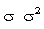)

• There are two classes of univariate descriptive statistics:
• measures of central tendency
• Central tendency gets at the "typical" or "most common" value in a set of values.
• measures of dispersion
• Dispersion tells how much spread or how much scattering there is around the central value.
• You use different measures of central tendency and dispersion for data scaled at different levels.
• This is because different levels of scaling produce different kinds of "numbers,"
• and they vary in the extent to which you can do arithmetic (addition, multiplication, etc.) on them.
• Central Tendency
• The mode is the most common category or value in the data.
• The median is the value at the midpoint of a rank-ordered list of all the values in a set of data.
• The mean is the arithmetic average of a set of values.
• The mode is the most common category or value in the data.
• If there are more red cars than any other colour, then red is the modal value of car colours.
• The mode is the only measure of central tendency that can be used with nominal data.
• The mode is not influenced by extreme values.
• The mode is sensitive only to the most frequently occurring score; it is insensitive to all other scores.
• The mode is of little value for non-categorical (e.g., continuous) data; it is used almost exclusively for discrete variables.
• The median is the value at the midpoint of a rank-ordered list of all the values in a set of data.
• If you have a large group of people line up in order from youngest to oldest, the age of the person in the middle of the line will be the median of the group's age.
• Half the values are above the median and half are below the median.
• If there are an odd number of scores, the median will be the center point in the rank-ordered list of points. (for example: if there are 5 people, the 3rd will be the median)
• If there are an even number of scores, the median will be the mean of the two centermost points. (for example, if there are 8 people, the median will be halfway between the 4th and 5th people, which is the mean of the 4th and 5th ones)
• Note that the median is not the midpoint of the range (the difference between the highest and lowest values).
• In the picture below, there are 14 women. The median is halfway between the 7th and 8th women.
• Half of the women (7) are above the median and half (7) are below the median.
•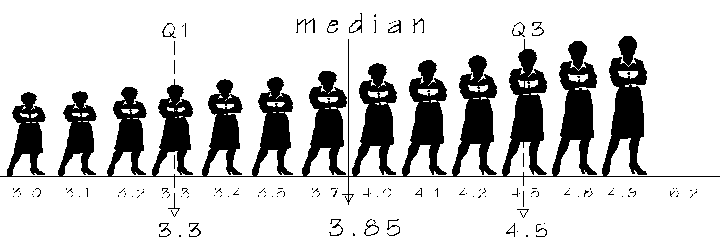• If you split the list of values into the half below the median and the half above the median (a "median split"), you could find the middle value of each half.
• These values (marked "Q1" and "Q3"), together with the median, divide the list of values into four parts called "quartiles"
• Each quartile contains 25% of all the cases in your data.
• The median is sometimes referred to as the "second quartile."
• Because the only thing it needs from your data is the order of the values, the median can be used for ordinal. Interval, or ratio scaled data --- all types of scaling except nominal.
• The median can be used for discrete or continuous variables.
• The median is not influenced by extreme values.
• The median is sensitive only to the value of the middle point or points; it is not sensitive to the values of all other points.
• The mean is the arithmetic average of a set of values.
• It is calculated by dividing the sum of all the values by the number of values.
• Because this requires addition, it can only be used with interval or ratio data.
• Since every value in a set of data affects the mean, the mean uses more of the information in the data than the median does.
• Extreme values have a disproportionately large effect on the mean.
• With the mean you see the first mathematical equation and symbols for this course.
•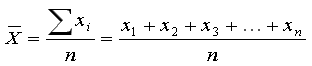• The symbol for the mean of a sample is X bar (pronounced "x-bar").
• The symbol for the mean of a population is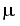(the lowercase Greek letter "mu").
• In the formula, X bar is the mean of all the values for the variable X in a set of data.
• The symbol that looks like a sideways "M" is a Greek uppercase sigma -- about as close as you can get in Greek to the letter "S" (as in "sum").
• It means "add up all the things that follow."
• The mean requires interval or ratio data.
• The mean is the preferred measure for interval or ratio data.
• The mean is generally not used for discrete variables.
• The mean is sensitive to all scores in a sample (every number in the data affects the mean), which makes it a more "powerful" measure than the median or mode.
• The mean's sensitivity to all scores also makes it sensitive to extreme values, which is why the median is used when there are extreme values.
• Dispersion
• If you look at almost any set of data, you will see that the individuals are not the same.
• For any variable, there will be many different scores or values -- some will be high; some low; some in the middle.
• The goal of most research is to describe or explain the variability in the data.
• Dispersion tells how scattered or spread out the values in the data are.
• The less spread out the values are, the more concentrated or clustered they will be . . .
• . . . and the more likely it is that there will be a "most common" or "typical" or "central" value.
• Also, the less dispersion there is, the more you can learn about the whole set of values by knowing its central value.
• The more spread out the values are, the more dispersion there will be, and the less you will know about the whole set if you know its central values.
• For nominal data, you are quite limited in what you can do.
• For nominal data, the only kind of comparison you can do with a pair of values is to see whether they are the same or different.
• For nominal data, you can only use a measure of dispersion that looks at the extent to which the values in the data are the same as or different from one another.
• The measure of dispersion that does this is the information-theoretic measure of uncertainty which you don't have to know how to do or interpret.
• For ordinal, interval, or ratio data, you can use the interquartile range (IQR) to measure dispersion.
• The IQR is the difference between the first and third quartiles in the data.
• If you remove the top 25% and the bottom 25% of all cases and then calculate the range of the remaining cases, you will get the IQR.
• You would say either "The IQR is 1.2" or, more likely, "The middle half of the sample have heights between 3.3 and 4.5."
•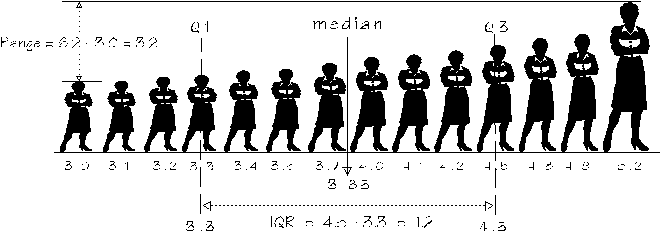• Variance
• All measures of dispersion are assessments of what you might call "variability"or "variety" -- the extent to which values in your data differ from one another.
• Variance is a particularly useful measure of variety or variability for interval-or ratio-scaled data.
• It is probably the most important statistical concept, and it is used in a very wide range of situations.
• The variance of a set of numbers is based on the distance between each value and the mean of all the values.
• You want to know how spread out your data is --how much variety there is in the set of values.
• A reasonable approach would be to ask how far from the mean the typical person is.
• If there is little variability, everyone will be close to the mean.
• If there is a lot of variability, people will be scattered all over the place with many a long distance from the mean.
• So it makes sense to starts with how far people are from the mean.
• The difference between a person's score and the mean is the person's deviation score:
•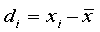• Deviation scores
•• If your original score is higher than the mean, your deviation score will be positive;
• if your original score is lower than the mean, your deviation score will be negative.
•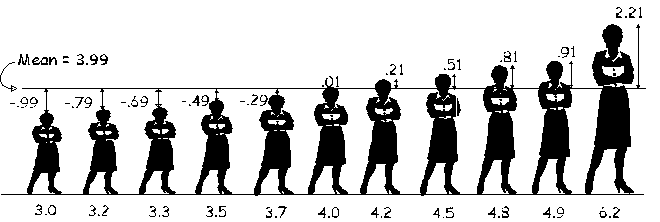• To get an idea of how far the typical person is from the mean, you might calculate the mean of the deviation scores
• This will tell you how far from the mean the typical person is.
• Here is a set of numbers, their mean, and their deviation scores:
 X x - mean deviation score 2 7 8 3 4 6 + 5 2 - 5 = 7 - 5 = 8 - 5 = 3 - 5 = 4 - 5 = 6 - 5 = 5 - 5 = -3 2 3 -2 -1 1 + 0 35 0 mean = 35 / 7 = 5

• Calculating the mean of the deviation scores seemed like a good way to get a measure of how much variety there is in a set of scores
• There is a problem with this, though, because the sum of the deviation scores is always zero . . .
• so the mean of the deviation scores will also always be zero
• It doesn't matter which data you do this with;
• the sum of the deviation scores is always zero, so the mean will also always be zero.
• This means that the mean deviation score is useless.
• It turns out that there is an easy way of dealing with negative deviation scores
• If you square a negative number, the result becomes positive
• So, if you square the deviation scores, the results will always be positive
• Instead of calculating the mean of the deviation scores, you calculate the mean of the squared deviation scores:
•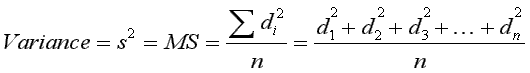• Because variance is the mean of the squared deviation scores, it is sometimes called the "mean square"
•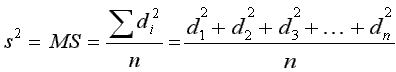• The closer the values in a set of data are to one another,
• the smaller the deviation scores will be,
• the smaller the squared deviation scores will be,
• the smaller the sum of the squared deviation scores will be,
• the smaller the mean of the squared deviation scores ("Mean Square" or "MS") will be, . . . . and . . . .
• the smaller the variance will be.
• The sum of the squared deviation scores in the numerator of the equation above is called the "sum of squares" (SS).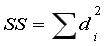• "Mean Square" is another name for "variance"
• The symbol for a population's variance is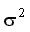• The letter in the symbol is a lowercase Greek sigma.
• Here is the equation for a population's variance:
•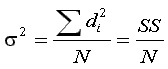• The symbol for a sample's variance is s2.
• Here is the equation for a sample's variance:
•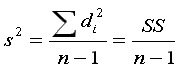• Since the variance is the mean of the squares of the deviation scores, it tells you what the "typical" squared deviation score is.
• Since you are probably more interested in the typical deviation score (how far the typical person is from the mean), you take the square root of the variance.
• Very roughly speaking, this is a way to undo the squaring you had to do for the variance.
• The square root of the variance is the Standard Deviation:
•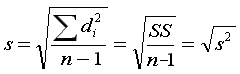•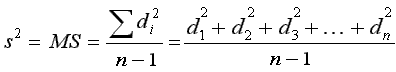• The variance, also known as the "mean square," is the mean of the squared deviation scores.
• For a population: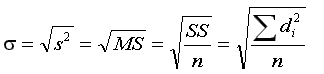• For a sample: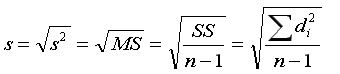• The standard deviation is the square root of the variance . . .
• so you can see that it is the square root of the mean of the squared deviation scores.
• This is why it is sometimes called the "root mean square"
• Six things about the standard deviation:
• While the variance is a measure of the overall amount of variability or spread around the mean . . .
• the standard deviation is a measure of the typical deviation from the mean.
• Like the variance and the mean, the standard deviation is sensitive to all scores.
• The symbol for a sample's standard deviation is a lowercase "s".
• You use "n-1" in the denominator if you have data for the members of the sample and are calculating the sample's standard deviation and using it to estimate the population's standard deviation.
• The symbol for population's standard deviation is a lowercase Greek sigma: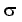• You use "n" in the denominator if you have data for every member of the population and are calculating the population's standard deviation.
• Are you trying to describe a sample or a population?
• To calculate variance or standard deviation for a population, you need to calculate the sum of the squares of the deviation scores for all members of the population.
• If you are working with a large population, you won't be able to get data from all members.
• Instead, you will have to use data from a sample to estimate the population values.
• To do this, use "n-1" in the denominator instead of "n".
•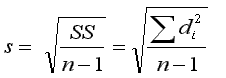vs.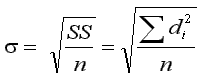• Standard Scores (or "z-scores")
• If an individual person's score is converted so it tells how far from the mean the person is, . . .
• it will become a relative score that will let you know how this person compares to the rest of the sample.
• The most common way of doing this is to calculate a standard score:
•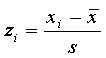• To get a person's z-score, subtract the mean from that person's score and divide the result by the standard deviation.
• The result tells you howfar away from the mean the person is, in terms of standard deviations --- how many standard deviations away from the mean the person is.
• The word "standard" in "standard score" is the same one as in "standard deviation."
• This is not a coincidence.
• To calculate standard scores, you divide the person's deviation score by the standard deviation.
• The equation to calculate a person's standard score is:
•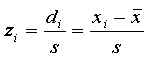• The subscript "i" tells which person you are doing this for.
• "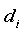" is person i's deviation score
• "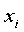" is person i's score on the variable
• "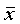" is the mean
• "s" is the standard deviation
• An example ...
• If the standard deviation is 2, the mean is 5, and your score is 9, your z-score would be:
•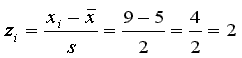•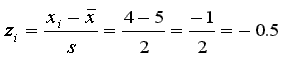• A positive z-score means that you are above the mean; a negative z-score means that you are below the mean.
• A person's z-score tells how far away from the mean that person's score is, in terms of standard deviations.
• If your z-score is 1.0, you are one standard deviation above the mean.
• If your z-score is 3.5, you are three and a half standard deviations above the mean.
• If your z-score is -2.5, you are two and a half standard deviations below the mean.
• Calculating Standard Deviation
• An alternative name for standard deviation, "root mean square," tells how to calculate it:
• the standard deviation is the square root of the mean of the squared deviation scores.
•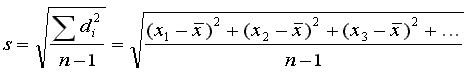• The deviation score (di) is the difference between the individual's score (Xi) and the mean(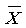).
• The equation above shows the additions, subtractions, and multiplications you have to do to calculate the standard deviation.
•• it works; and
• you can remember it if you can remember "root mean square."

• The bad thing about it is that it involves a lot of work:
• You have to calculate the mean.
• You have to subtract the mean from every value on the list.
• You have to square each difference.
• You have to calculate the sum of the squares.
• Finally, you divide by n - 1 and take the square root.
• Here is an example worked out with this original equation:
 xi di di2 7 -2.625 6.890625 12 2.375 5.640625 9 -0.625 0.390625 11 1.375 1.890625 8 -1.625 2.640625 6 -3.625 13.140625 10 0.375 0.140625 + 14 4.375 + 19.140625 77 49.875000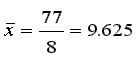so 7 - 9.625 = -2.625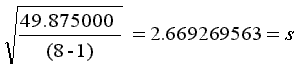• Here is a different equation for standard deviation, called the "computational form" because it is much easier to use:

• It is easier because you don't have to calculate the mean or the deviation scores:

•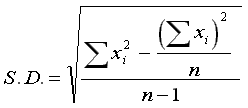• With this equation, you only have to calculate two easy sums and one difference.
•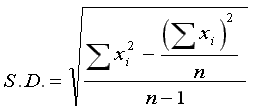• 1) First, calculate the sum of the squares of the original scores: xi2
• 2) Then calculate the sum of the original scores and square it: xi � xi = xi2
• 3) Divide the result of step 2 by n, the sample size.
• 4) Now subtract the result of step 3 from the first sum.
• 5) Divide the result of step 4 by your sample size minus 1.
• 6) Take the square root. Voila!

 xi xi2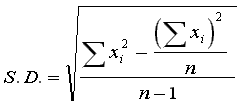7 49 12 144 9 81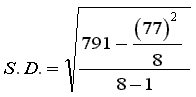11 121 8 64 6 36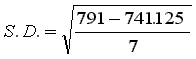10 100 + 14 + 196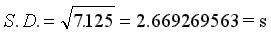77 791 77 � 77 = 5929 5929 � 8 = 741.125 791 - 741.125 = 49.875 variance = 49.875/7 = 7.125 = s2 std. dev. = square root of variance = 2.669269563 = s

• The result is exactly the same as if you calculated the mean, the deviation scores, the squares of the deviation scores, the sum of the squares, except . . . .

• With the computational method, you do a lot less work.
• You only need to add up all the scores for one total and then add up the squares of the scores for the second total.
• The rest is easy.
• The table below shows a comparison of the amount of work you have to do with the two methods for samples containing 7 and 50 cases.

 original method computational form n = 8 n = 50 n = 8 n = 50 additions 16 100 16 100 subtractions 9 51 2 2 multiplications 8 50 9 51 divisions 2 2 2 2 square roots 1 1 1 1 total 36 204 30 156

• The numbers in the table actually underestimate the difference in the amount of work.
• This is because the original method usually requires working with ugly numbers -- numbers involving decimals like 27.3841, 4.1428571428, etc.
• These numbers result from subtracting the mean from the original scores to calculate deviation scores.
• When you square the deviation scores, the numbers get even uglier, increasing the amount of time it takes to do the work, and requiring more rounding, which introduces rounding errors.
• In comparison, almost all of the numbers you use with the computational form are whole numbers.
• The computational form takes less work, and there is less rounding error.
• The standard deviation is the most common measure of dispersion when the data is scaled at the interval or ratio level.
• It is a measure of how spread out the members of a sample are from one another . . .
• . . . in particular, how far the typical value is from the mean.
• This means that that knowing the standard deviation allows you to know how good an estimate of central tendency the mean is.
• If the standard deviation is small, all the values are close to the mean, so the mean is a pretty good description of the "typical" value.
• When you calculate the sample's standard deviation with (n-1) in the denominator . . .
• you can use it as an estimate of the population's standard deviation.
• When you combine the mean with the standard deviation in the calculation of z-scores (for a normally distributed variable), you can tell where an individual is in the distribution relative to the other members.
• For example, if your z- score is 2.52, you are above 97.5% of everyone else in the sample.
• If your z-score is 1.0, about 16% of the other people are above you.